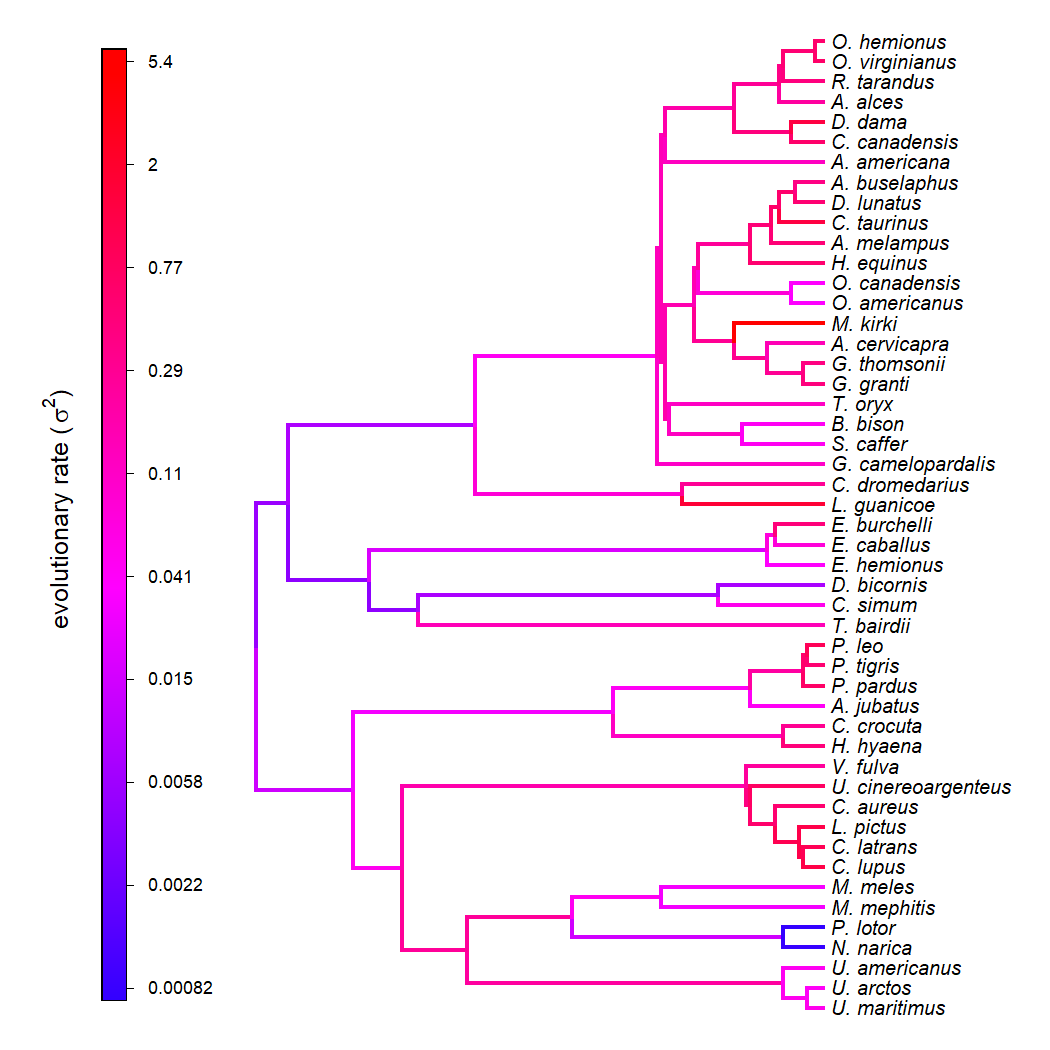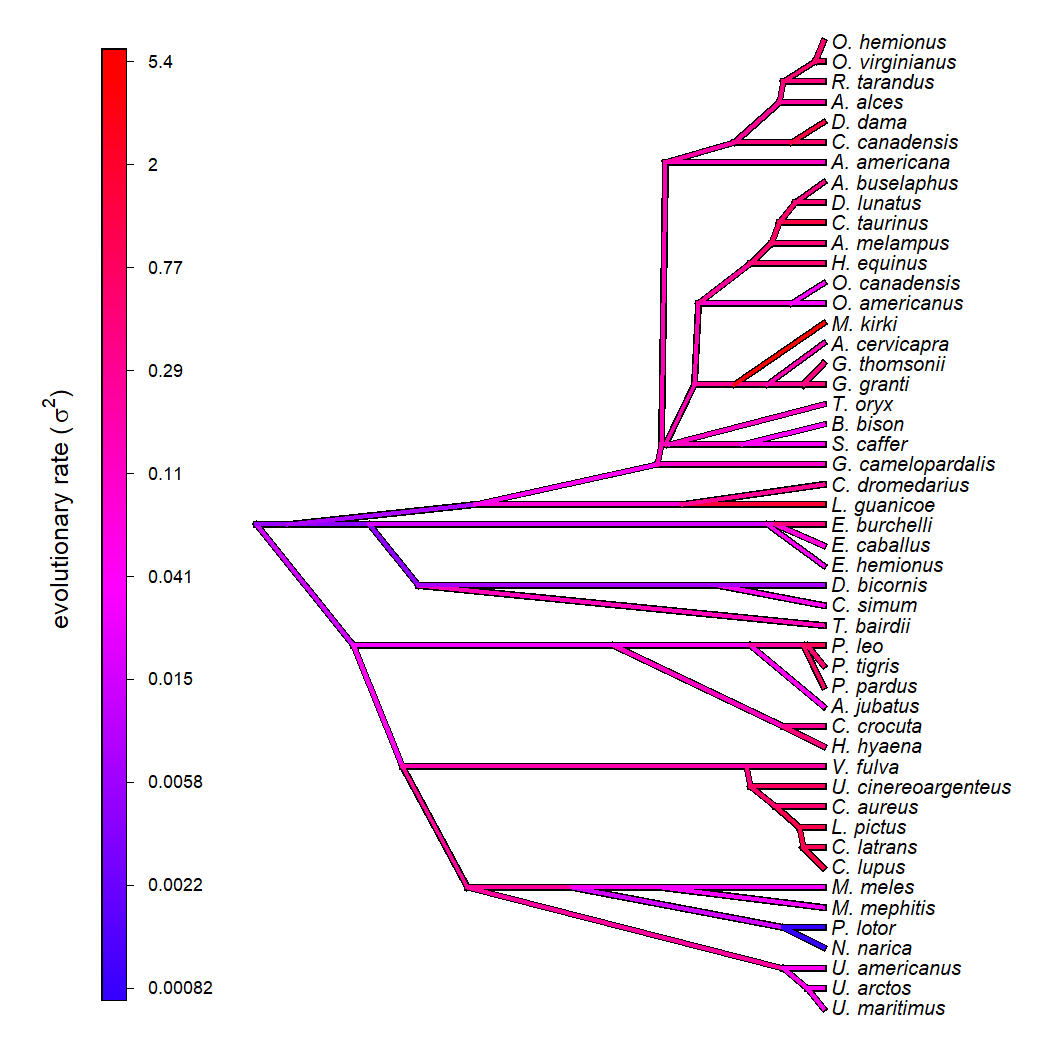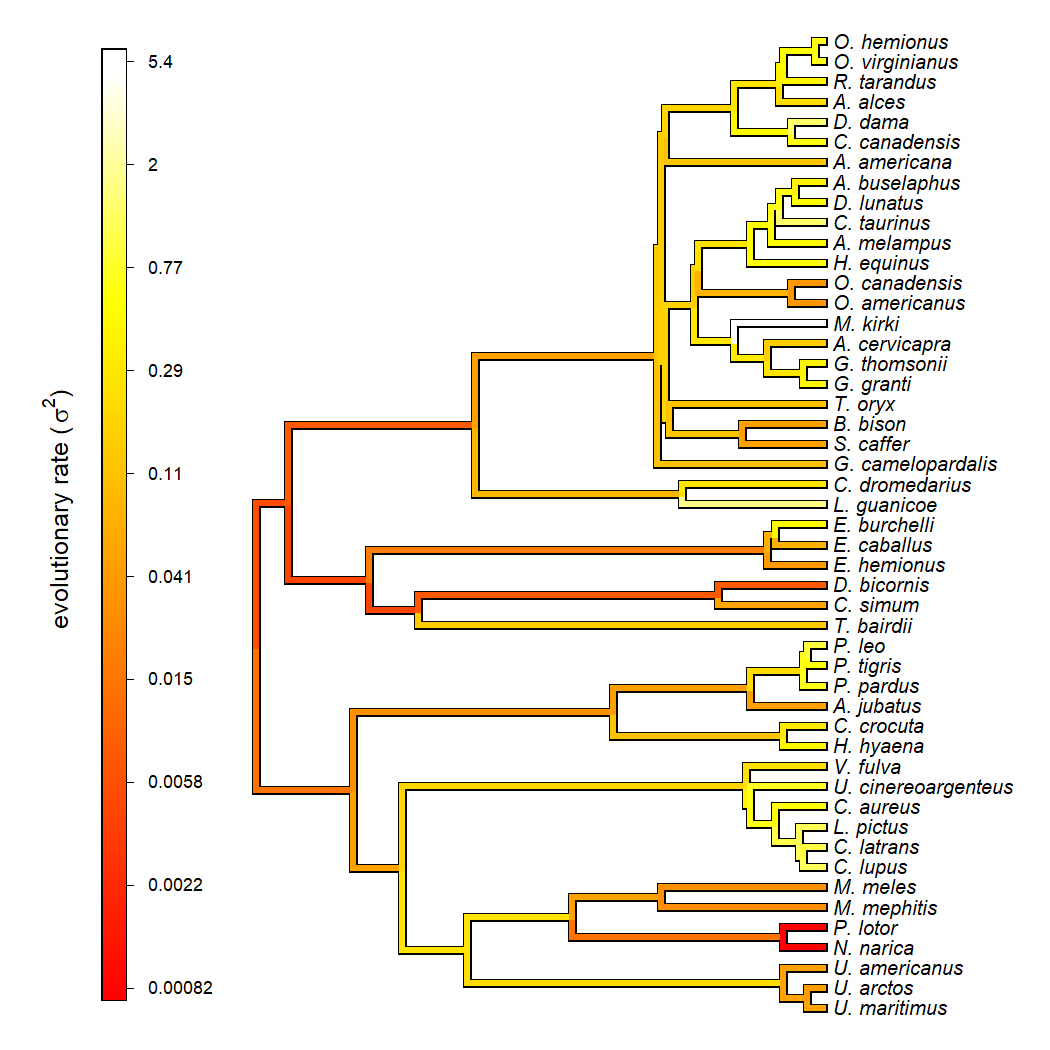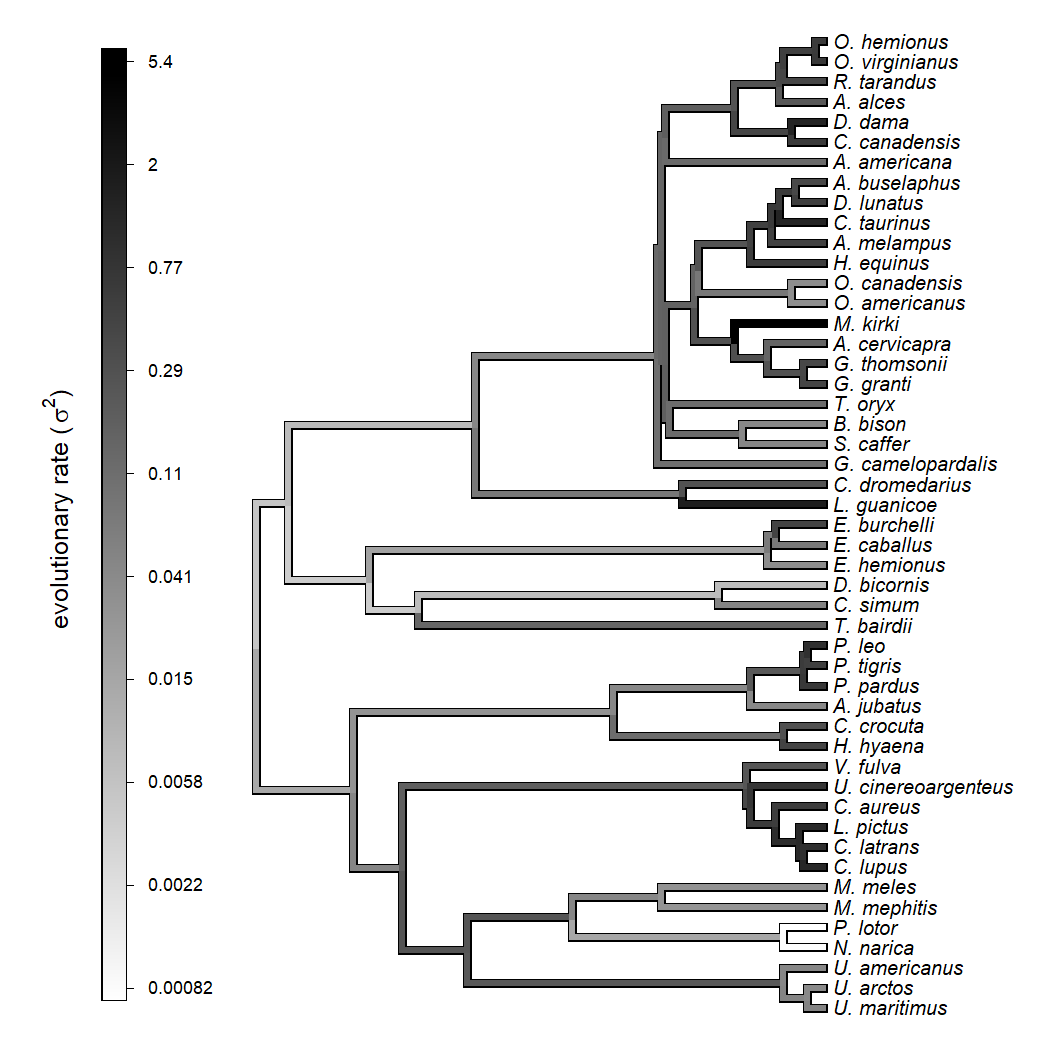## Monday, April 5, 2021

### Updated plotting method for variable-rate penalized likelihood model in phytools

Inspired by comments from my friend & collaborator Lars Schmitz, I just pushed some updates to the variable rate Brownian motion penalized likelihood method that I blogged about here last week.

The idea of this approach, remember, is to fit a variable-rate Brownian motion model to our tree and data, in which the rate parameter itself (σ2) evolves from branch to branch in the tree (by a Brownian process on the log scale). Because this model would be non-identifiable if we allowed σ2 for the evolutionary rate to assume any value, we use a term (the probability of the rates under our model), and a user-specified penalty coefficient (λ), to determine how much σ2 should be permitted to change through time and among lineages. Larger values of the penalty term λ result in less changes; whereas lower values of λ permit σ2 to evolve from edge to edge on the phylogeny with relatively little cost.

In this update the main thing that I did was add much more user control of the color schema used for plotting our fitted object from the `multirateBM` function, as well as more options for the style of the plotted tree.

Here's a quick example using log-transformed home range size for mammals. These data come from Garland et al. (1992).

``````library(phytools)
``````
``````## Loading required package: ape
``````
``````## Loading required package: maps
``````
``````data(mammal.tree)
data(mammal.data)
lnHomeRange<-setNames(log(mammal.data\$homeRange),
rownames(mammal.data))
fit.mammal<-multirateBM(mammal.tree,lnHomeRange,
lambda=1.0)
``````
``````## Beginning optimization....
## Optimization iteration 1. Using "Nelder-Mead" optimization method.
## Optimization iteration 2. Using "BFGS" optimization method.
## Done optimization.
``````
``````fit.mammal
``````
``````## Multi-rate Brownian model using multirateBM.
##
## Fitted rates:
##  U._maritimus U._arctos U._americanus N._narica P._lotor M._mephitis M._meles
##      0.036776  0.026262      0.029172  0.000897 0.000818    0.021497 0.023634
##  C._lupus
##  0.546833 ....
##
## lambda penalty term: 1
## log-likelihood:  -79.564297
## AIC:  355.128593
##
## R thinks it has found a solution.
``````

Now let's make our plot using the default settings. When I do it, I'll also return the plotted object to the user as follows:

``````object<-plot(fit.mammal,ftype="i",fsize=0.8)
````````````object
``````
``````## Object of class "multirateBM_plot" containing:
##
## (1) A phylogenetic tree with 49 tips and 48 internal nodes.
##
## (2) A mapped set of Brownian evolution rates.
``````

Now I'm going to re-plot this object in a different style:

``````plot(object,type="cladogram",ftype="i",fsize=0.8,
nodes="inner",outline=TRUE)
``````That's kind of neat, right.

Now let's change the color too. We'll do this using the phytools generic function `setMap` as follows:

``````object<-setMap(object,colors=heat.colors(n=1000))
plot(object,ftype="i",fsize=0.8,outline=TRUE,
lwd=4)
``````We can also use a totally arbitrary gradient if we want. Here's a grey-scale example:

``````object<-setMap(object,colors=c("white","black"))
plot(object,ftype="i",fsize=0.8,outline=TRUE,
lwd=4)
``````That's pretty cool.

For those interested in such things, some of the things that you'll notice in the source code of this update are: (1) a new object class (`"multirateBM_plot"`) containing the plot object, rather than the fitted model; and (2) use of `do.call` to call the plot methods (mostly, `phytools::plotSimmap`) internally. `do.call` is a very handy function when we want to allow users to have a lot of flexibility in passing their own argument values to internally used functions, but some values for these arguments would break our function. Check it out!